# Algebra II : Direct Proportionality

## Example Questions

1 3 Next →

### Example Question #21 : Basic Single Variable Algebra

Suppose a runner's distance is directly proportional to her time.  If the runner completes 6 miles in 70 minutes, how many minutes did it take the runner to run 4 miles?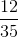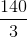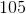Explanation:

Write the equation for direct proportionality.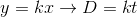Substitute the distance and time to solve for theconstant.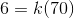Divide by 70 on both sides.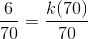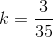Substitute this value back to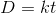.

The equation becomes: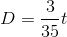Substitute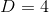to determine how long it took the runner to run 4 miles.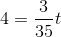Multiply by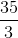on both sides to isolate the time variable.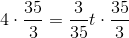Simplify both sides.

The answer is:### Example Question #22 : Direct Proportionality

The distance of a cyclist is directly proportional to the time he has traveled. Suppose he has traveled 12 miles in 1.5 hours. How far does he travel in a half hour?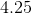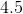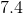Explanation:

Write the equation for direct proportionality.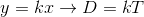Substitute the distance and time given to solve for the constant of proportionality,.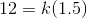Divide by 1.5 on both sides.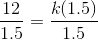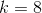Write the equation.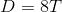Substitute half an hour for the time to determine the distance the biker has traveled.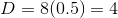The biker traveled four miles in a half hour.

The answer is:1 3 Next →

### All Algebra II Resources